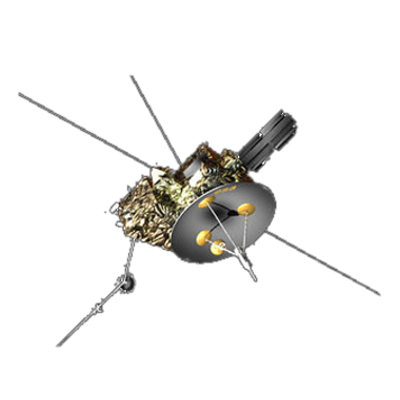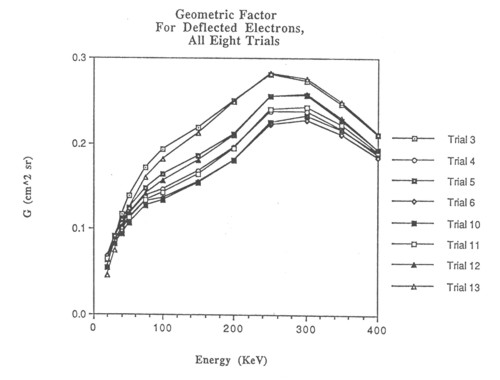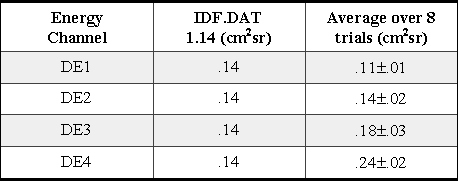ULYSSES

Ulysses HISCALE Data Analysis Handbook

## Appendix 9  Geometric Factor Study for the Deflected and Unscattered Electrons of HISCALE (Buckley MS Thesis)

Figure 9-14 Plots of G vs. E for all eight trials. Note that all curves follow a general trend.During the course of this study, a total of three different geometries and four magnetic fields were used.  The first geometry adopted was missing a notch that was later filed in the actual flight instrument.  Results for this are not discussed in this paper.  The second and third geometries are both similar versions of the geometry with the notch included.  For each of the later two geometries four slightly different magnetic fields were used to determine the geometrical factors.  The resulting plots of G vs. E are shown in Figure A9-14, and Table A9-3 gives the geometric factors for the four energy channels averaged over all eight trials.

Table A9-3 Geometric factors for deflected electrons combined average of notch one and two.The current values used in the production program of the HISCALE instrument are summarized in a data file IDF.DAT.  Also listed in Table A9-3 are the IDF.DAT values of the geometric factors for the deflected electrons.  As one observes from the table, there are certainly some discrepancies between the two sets of values.  These and other problems are discussed below.